# 5.6 Rational functions  (Page 11/16)

 Page 11 / 16

$f\left(x\right)=\frac{x}{2x+1}$

Local behavior: $\text{\hspace{0.17em}}x\to -{\frac{1}{2}}^{+},f\left(x\right)\to -\infty ,x\to -{\frac{1}{2}}^{-},f\left(x\right)\to \infty \text{\hspace{0.17em}}$

End behavior: $\text{\hspace{0.17em}}x\to ±\infty ,f\left(x\right)\to \frac{1}{2}$

$f\left(x\right)=\frac{2x}{x-6}$

$f\left(x\right)=\frac{-2x}{x-6}$

Local behavior: $\text{\hspace{0.17em}}x\to {6}^{+},f\left(x\right)\to -\infty ,x\to {6}^{-},f\left(x\right)\to \infty ,\text{\hspace{0.17em}}$ End behavior: $\text{\hspace{0.17em}}x\to ±\infty ,f\left(x\right)\to -2$

$f\left(x\right)=\frac{{x}^{2}-4x+3}{{x}^{2}-4x-5}$

$f\left(x\right)=\frac{2{x}^{2}-32}{6{x}^{2}+13x-5}$

Local behavior: $\text{\hspace{0.17em}}x\to -{\frac{1}{3}}^{+},f\left(x\right)\to \infty ,x\to -{\frac{1}{3}}^{-},\text{\hspace{0.17em}}$ $f\left(x\right)\to -\infty ,x\to {\frac{5}{2}}^{-},f\left(x\right)\to \infty ,x\to {\frac{5}{2}}^{+},f\left(x\right)\to -\infty$

End behavior: $x\to ±\infty ,\phantom{\rule{0.2em}{0ex}}f\left(x\right)\to \frac{1}{3}$

For the following exercises, find the slant asymptote of the functions.

$f\left(x\right)=\frac{24{x}^{2}+6x}{2x+1}$

$f\left(x\right)=\frac{4{x}^{2}-10}{2x-4}$

$y=2x+4$

$f\left(x\right)=\frac{81{x}^{2}-18}{3x-2}$

$f\left(x\right)=\frac{6{x}^{3}-5x}{3{x}^{2}+4}$

$y=2x$

$f\left(x\right)=\frac{{x}^{2}+5x+4}{x-1}$

## Graphical

For the following exercises, use the given transformation to graph the function. Note the vertical and horizontal asymptotes.

The reciprocal function shifted up two units.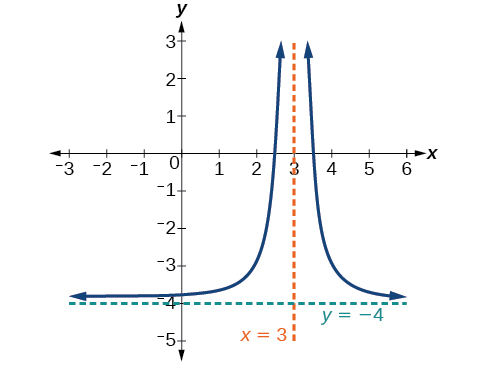The reciprocal function shifted down one unit and left three units.

The reciprocal squared function shifted to the right 2 units.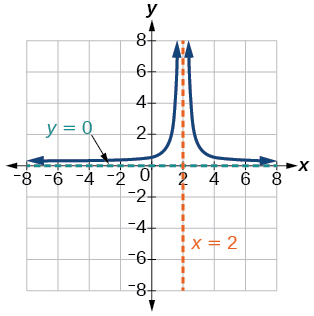The reciprocal squared function shifted down 2 units and right 1 unit.

For the following exercises, find the horizontal intercepts, the vertical intercept, the vertical asymptotes, and the horizontal or slant asymptote of the functions. Use that information to sketch a graph.

$p\left(x\right)=\frac{2x-3}{x+4}$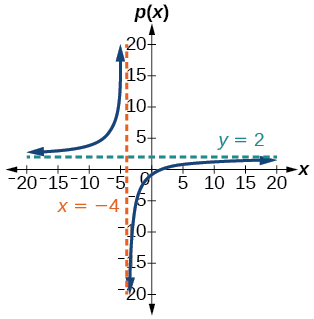$q\left(x\right)=\frac{x-5}{3x-1}$

$s\left(x\right)=\frac{4}{{\left(x-2\right)}^{2}}$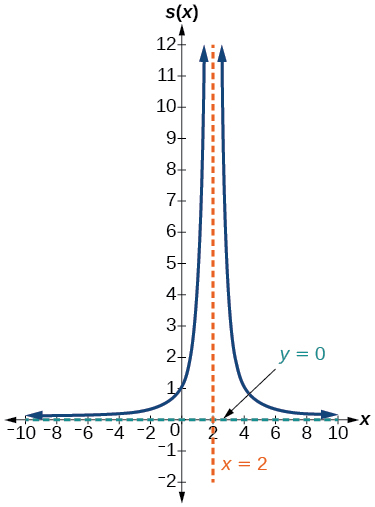$r\left(x\right)=\frac{5}{{\left(x+1\right)}^{2}}$

$f\left(x\right)=\frac{3{x}^{2}-14x-5}{3{x}^{2}+8x-16}$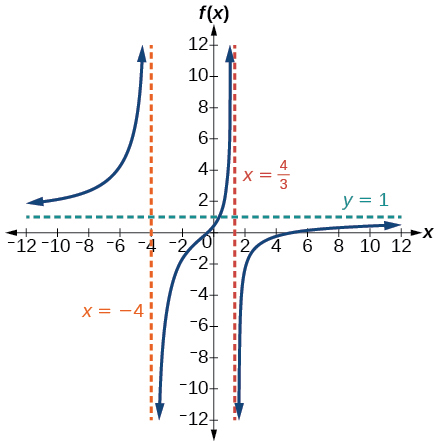$g\left(x\right)=\frac{2{x}^{2}+7x-15}{3{x}^{2}-14+15}$

$a\left(x\right)=\frac{{x}^{2}+2x-3}{{x}^{2}-1}$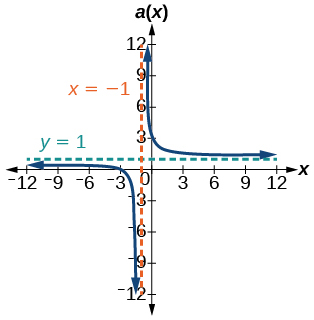$b\left(x\right)=\frac{{x}^{2}-x-6}{{x}^{2}-4}$$k\left(x\right)=\frac{2{x}^{2}-3x-20}{x-5}$

$w\left(x\right)=\frac{\left(x-1\right)\left(x+3\right)\left(x-5\right)}{{\left(x+2\right)}^{2}\left(x-4\right)}$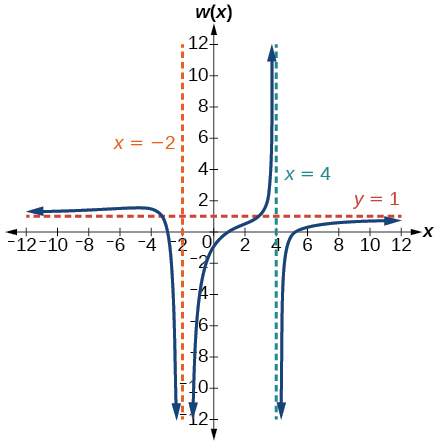$z\left(x\right)=\frac{{\left(x+2\right)}^{2}\left(x-5\right)}{\left(x-3\right)\left(x+1\right)\left(x+4\right)}$

For the following exercises, write an equation for a rational function with the given characteristics.

Vertical asymptotes at $\text{\hspace{0.17em}}x=5\text{\hspace{0.17em}}$ and $\text{\hspace{0.17em}}x=-5,\text{\hspace{0.17em}}$ x -intercepts at $\text{\hspace{0.17em}}\left(2,0\right)\text{\hspace{0.17em}}$ and $\text{\hspace{0.17em}}\left(-1,0\right),\text{\hspace{0.17em}}$ y -intercept at $\text{\hspace{0.17em}}\left(0,4\right)$

$y=50\frac{{x}^{2}-x-2}{{x}^{2}-25}$

Vertical asymptotes at $\text{\hspace{0.17em}}x=-4\text{\hspace{0.17em}}$ and $\text{\hspace{0.17em}}x=-1,\text{\hspace{0.17em}}$ x- intercepts at $\text{\hspace{0.17em}}\left(1,0\right)\text{\hspace{0.17em}}$ and $\text{\hspace{0.17em}}\left(5,0\right),\text{\hspace{0.17em}}$ y- intercept at $\text{\hspace{0.17em}}\left(0,7\right)$

Vertical asymptotes at $\text{\hspace{0.17em}}x=-4\text{\hspace{0.17em}}$ and $\text{\hspace{0.17em}}x=-5,\text{\hspace{0.17em}}$ x -intercepts at $\text{\hspace{0.17em}}\left(4,0\right)\text{\hspace{0.17em}}$ and $\text{\hspace{0.17em}}\left(-6,0\right),\text{\hspace{0.17em}}$ Horizontal asymptote at $\text{\hspace{0.17em}}y=7$

$y=7\frac{{x}^{2}+2x-24}{{x}^{2}+9x+20}$

Vertical asymptotes at $\text{\hspace{0.17em}}x=-3\text{\hspace{0.17em}}$ and $\text{\hspace{0.17em}}x=6,\text{\hspace{0.17em}}$ x -intercepts at $\text{\hspace{0.17em}}\left(-2,0\right)\text{\hspace{0.17em}}$ and $\text{\hspace{0.17em}}\left(1,0\right),\text{\hspace{0.17em}}$ Horizontal asymptote at $\text{\hspace{0.17em}}y=-2$

Vertical asymptote at $\text{\hspace{0.17em}}x=-1,\text{\hspace{0.17em}}$ Double zero at $\text{\hspace{0.17em}}x=2,\text{\hspace{0.17em}}$ y -intercept at $\text{\hspace{0.17em}}\left(0,2\right)$

$y=\frac{1}{2}\frac{{x}^{2}-4x+4}{x+1}$

Vertical asymptote at $\text{\hspace{0.17em}}x=3,\text{\hspace{0.17em}}$ Double zero at $\text{\hspace{0.17em}}x=1,\text{\hspace{0.17em}}$ y -intercept at $\text{\hspace{0.17em}}\left(0,4\right)$

For the following exercises, use the graphs to write an equation for the function.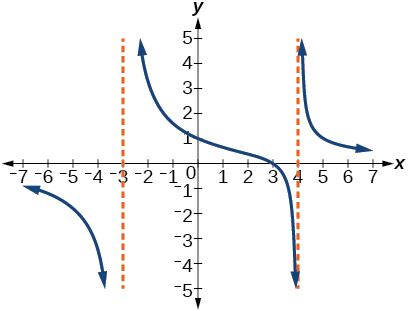$y=4\frac{x-3}{{x}^{2}-x-12}$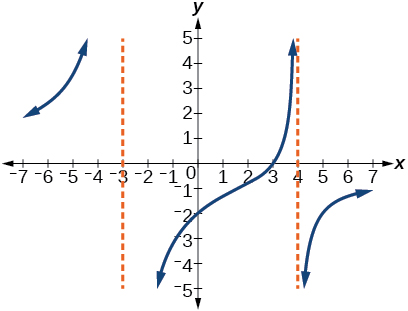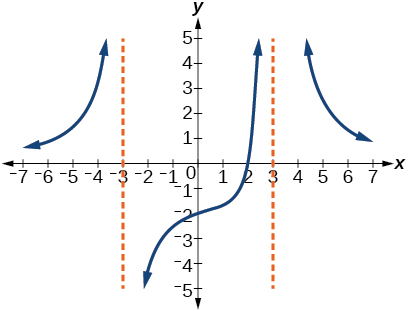$y=-9\frac{x-2}{{x}^{2}-9}$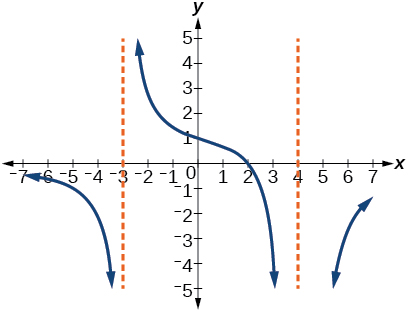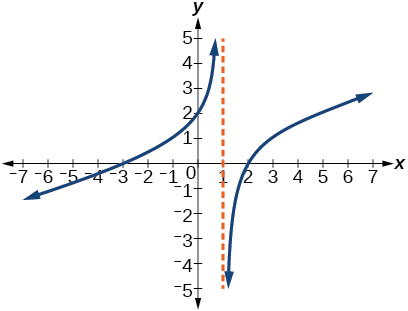$y=\frac{1}{3}\frac{{x}^{2}+x-6}{x-1}$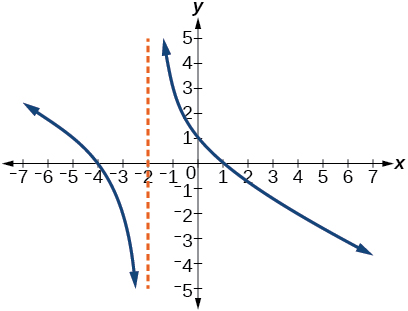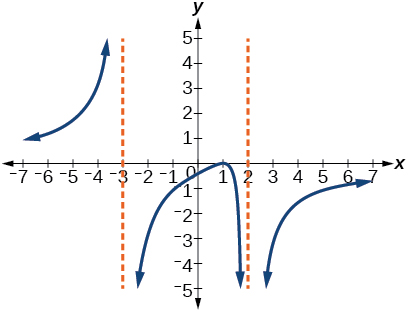$y=-6\frac{{\left(x-1\right)}^{2}}{\left(x+3\right){\left(x-2\right)}^{2}}$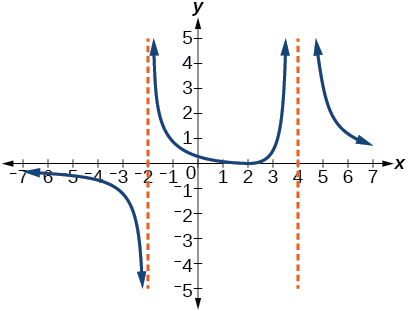## Numeric

For the following exercises, make tables to show the behavior of the function near the vertical asymptote and reflecting the horizontal asymptote

$f\left(x\right)=\frac{1}{x-2}$

 $x$ 2.01 2.001 2.0001 1.99 1.999 $y$ 100 1,000 10,000 –100 –1,000
$x$ 10 100 1,000 10,000 100,000
$y$ .125 .0102 .001 .0001 .00001

Vertical asymptote $\text{\hspace{0.17em}}x=2,\text{\hspace{0.17em}}$ Horizontal asymptote $\text{\hspace{0.17em}}y=0$

$f\left(x\right)=\frac{x}{x-3}$

$f\left(x\right)=\frac{2x}{x+4}$

 $x$ –4.1 –4.01 –4.001 –3.99 –3.999 $y$ 82 802 8,002 –798 –7998
 $x$ 10 100 1,000 10,000 100,000 $y$ 1.4286 1.9331 1.992 1.9992 1.999992

Vertical asymptote $\text{\hspace{0.17em}}x=-4,\text{\hspace{0.17em}}$ Horizontal asymptote $\text{\hspace{0.17em}}y=2$

$f\left(x\right)=\frac{2x}{{\left(x-3\right)}^{2}}$

$f\left(x\right)=\frac{{x}^{2}}{{x}^{2}+2x+1}$

 $x$ –.9 –.99 –.999 –1.1 –1.01 $y$ 81 9,801 998,001 121 10,201
 $x$ 10 100 1,000 10,000 100,000 $y$ 0.82645 0.9803 .998 .9998

Vertical asymptote $\text{\hspace{0.17em}}x=-1,\text{\hspace{0.17em}}$ Horizontal asymptote $\text{\hspace{0.17em}}y=1$

## Technology

For the following exercises, use a calculator to graph $\text{\hspace{0.17em}}f\left(x\right).\text{\hspace{0.17em}}$ Use the graph to solve $\text{\hspace{0.17em}}f\left(x\right)>0.$

$f\left(x\right)=\frac{2}{x+1}$

$f\left(x\right)=\frac{4}{2x-3}$

$\left(\frac{3}{2},\infty \right)$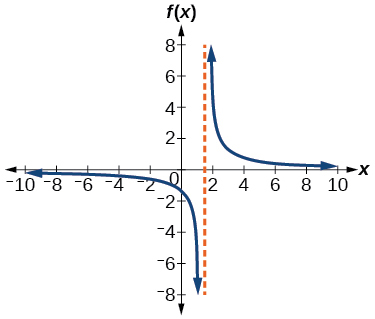$f\left(x\right)=\frac{2}{\left(x-1\right)\left(x+2\right)}$

$f\left(x\right)=\frac{x+2}{\left(x-1\right)\left(x-4\right)}$

$\left(-2,1\right)\cup \left(4,\infty \right)$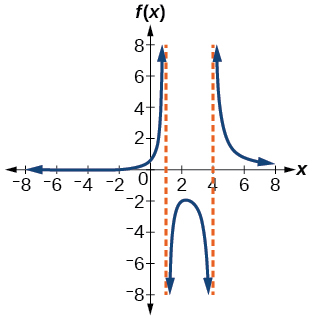$f\left(x\right)=\frac{{\left(x+3\right)}^{2}}{{\left(x-1\right)}^{2}\left(x+1\right)}$

## Extensions

For the following exercises, identify the removable discontinuity.

$f\left(x\right)=\frac{{x}^{2}-4}{x-2}$

$\left(2,4\right)$

$f\left(x\right)=\frac{{x}^{3}+1}{x+1}$

$f\left(x\right)=\frac{{x}^{2}+x-6}{x-2}$

$\left(2,5\right)$

$f\left(x\right)=\frac{2{x}^{2}+5x-3}{x+3}$

$f\left(x\right)=\frac{{x}^{3}+{x}^{2}}{x+1}$

$\left(–1,\text{1}\right)$

## Real-world applications

For the following exercises, express a rational function that describes the situation.

A large mixing tank currently contains 200 gallons of water, into which 10 pounds of sugar have been mixed. A tap will open, pouring 10 gallons of water per minute into the tank at the same time sugar is poured into the tank at a rate of 3 pounds per minute. Find the concentration (pounds per gallon) of sugar in the tank after $\text{\hspace{0.17em}}t\text{\hspace{0.17em}}$ minutes.

A large mixing tank currently contains 300 gallons of water, into which 8 pounds of sugar have been mixed. A tap will open, pouring 20 gallons of water per minute into the tank at the same time sugar is poured into the tank at a rate of 2 pounds per minute. Find the concentration (pounds per gallon) of sugar in the tank after $\text{\hspace{0.17em}}t\text{\hspace{0.17em}}$ minutes.

$C\left(t\right)=\frac{8+2t}{300+20t}$

For the following exercises, use the given rational function to answer the question.

The concentration $\text{\hspace{0.17em}}C\text{\hspace{0.17em}}$ of a drug in a patient’s bloodstream $\text{\hspace{0.17em}}t\text{\hspace{0.17em}}$ hours after injection in given by $\text{\hspace{0.17em}}C\left(t\right)=\frac{2t}{3+{t}^{2}}.\text{\hspace{0.17em}}$ What happens to the concentration of the drug as $\text{\hspace{0.17em}}t\text{\hspace{0.17em}}$ increases?

The concentration $\text{\hspace{0.17em}}C\text{\hspace{0.17em}}$ of a drug in a patient’s bloodstream $\text{\hspace{0.17em}}t\text{\hspace{0.17em}}$ hours after injection is given by $\text{\hspace{0.17em}}C\left(t\right)=\frac{100t}{2{t}^{2}+75}.\text{\hspace{0.17em}}$ Use a calculator to approximate the time when the concentration is highest.

After about 6.12 hours.

For the following exercises, construct a rational function that will help solve the problem. Then, use a calculator to answer the question.

An open box with a square base is to have a volume of 108 cubic inches. Find the dimensions of the box that will have minimum surface area. Let $\text{\hspace{0.17em}}x\text{\hspace{0.17em}}$ = length of the side of the base.

A rectangular box with a square base is to have a volume of 20 cubic feet. The material for the base costs 30 cents/ square foot. The material for the sides costs 10 cents/square foot. The material for the top costs 20 cents/square foot. Determine the dimensions that will yield minimum cost. Let $\text{\hspace{0.17em}}x\text{\hspace{0.17em}}$ = length of the side of the base.

$A\left(x\right)=50{x}^{2}+\frac{800}{x}.\text{\hspace{0.17em}}$ 2 by 2 by 5 feet.

A right circular cylinder has volume of 100 cubic inches. Find the radius and height that will yield minimum surface area. Let $\text{\hspace{0.17em}}x\text{\hspace{0.17em}}$ = radius.

A right circular cylinder with no top has a volume of 50 cubic meters. Find the radius that will yield minimum surface area. Let $\text{\hspace{0.17em}}x\text{\hspace{0.17em}}$ = radius.

$A\left(x\right)=\pi {x}^{2}+\frac{100}{x}.\text{\hspace{0.17em}}$ Radius = 2.52 meters.

A right circular cylinder is to have a volume of 40 cubic inches. It costs 4 cents/square inch to construct the top and bottom and 1 cent/square inch to construct the rest of the cylinder. Find the radius to yield minimum cost. Let $\text{\hspace{0.17em}}x\text{\hspace{0.17em}}$ = radius.

#### Questions & Answers

write down the polynomial function with root 1/3,2,-3 with solution
if A and B are subspaces of V prove that (A+B)/B=A/(A-B)
write down the value of each of the following in surd form a)cos(-65°) b)sin(-180°)c)tan(225°)d)tan(135°)
Prove that (sinA/1-cosA - 1-cosA/sinA) (cosA/1-sinA - 1-sinA/cosA) = 4
what is the answer to dividing negative index
In a triangle ABC prove that. (b+c)cosA+(c+a)cosB+(a+b)cisC=a+b+c.
give me the waec 2019 questions
the polar co-ordinate of the point (-1, -1)
prove the identites sin x ( 1+ tan x )+ cos x ( 1+ cot x )= sec x + cosec x
tanh`(x-iy) =A+iB, find A and B
B=Ai-itan(hx-hiy)
Rukmini
what is the addition of 101011 with 101010
If those numbers are binary, it's 1010101. If they are base 10, it's 202021.
Jack
extra power 4 minus 5 x cube + 7 x square minus 5 x + 1 equal to zero
the gradient function of a curve is 2x+4 and the curve passes through point (1,4) find the equation of the curve
1+cos²A/cos²A=2cosec²A-1
test for convergence the series 1+x/2+2!/9x3ByByByBy